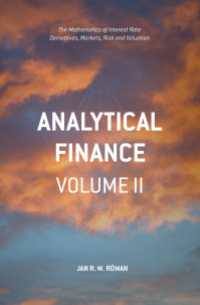## 電子書籍詳細Analytical Finance: Volume II : The Mathematics of Interest Rate Derivatives, Markets, Risk and Valuation . 1st ed. 2017

Röman, Jan R. M.

Palgrave Macmillan 2017/11
XXXI, 728 p. 141 illus.

ISBN: 9783319525839
eISBN: 9783319525846
KNPID: EY00221289

ファイルフォーマット:
ファイルサイズ:
デバイス:ご購入を希望される方は、下のリンクをクリックしてください。

### Full Description

Analytical Finance is a comprehensive introduction to the financial engineering of equity and interest rate instruments for financial markets. Developed from notes from the author’s many years in quantitative risk management and modeling roles, and then for the Financial Engineering course at Mälardalen University, it provides exhaustive coverage of vanilla and exotic mathematical finance applications for trading and risk management, combining rigorous theory with real market application.

Coverage includes:

• Date arithmetic’s, quote types of interest rate instruments
• The interbank market and reference rates, including negative rates
• Valuation and modeling of IR instruments; bonds, FRN, FRA, forwards, futures, swaps, CDS, caps/floors and others
• Bootstrapping and how to create interest rate curves from prices of traded instruments
• Risk measures of IR instruments
• The term structure equation, martingale measures and stochastic processes of interest rates; Vasicek, Ho-Lee, Hull-While, CIR
• Numerical models; Black-Derman-Toy and forward induction using Arrow-Debreu prices and Newton–Raphson in 2 dimension
• The Heath-Jarrow-Morton framework
• Forward measures and general option pricing models
• Black log-normal and, normal model for derivatives, market models and managing exotics instruments
• Pricing before and after the financial crisis, collateral discounting, multiple curve framework, cheapest-to-deliver curves, CVA, DVA and FVA

Pricing via Arbitrage

The Central Limit Theorem

The Binomial model

More on Binomial models

Finite difference methods

Value-at-Risk - VaR

Introduction to probability theory

Stochastic integration

Partial parabolic differential equations and Feynman-Kač

The Black-Scholes-Merton model

American versus European options

Analytical pricing formulas for American options

Poisson processes and jump diffusion

Diffusion models in general

Hedging

Exotic Options

Volatility